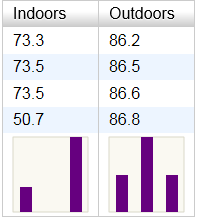## Tuesday, June 18, 2013

### Histogram thumbnails inside YUI3 data grids

Sparklines are tiny graphs added inline to data tables or lists. Sparklines have no units or gridlines; the purpose is just to quickly convey the shape of the data, which is usually presented adjacent.

A powerful variant on sparklines are sparkline histograms, or "sparkgrams" as I like to call them. Sparkgrams can quickly and compactly convey data distributions: outliers, right/left skew, normal vs. uniform distribution, etc. Because they are small, they can be included right inside a data table for every column or row. Such a presentation is useful either for completely known data when embarking upon data analysis, or for newly received data of a known data type in, for example, a manufacturing quality control scenario.

Below is an example of how it might look. The first column demonstrates an outlier, while the second column conveys a normal distribution.Below is the YUI3 code to render the above.

```<!DOCTYPE html>
<script src="yui/build/yui/yui-min.js"></script>
<script>
YUI().use('datatable', 'charts', function(Y) {
var fmt = function(o) {
if (o.value.substring && o.value.substring(0,5) === "chart")
o.cell.set("innerHTML", "<div id='" + o.value +
"' style='width:75px;height:75px'></div>");
else
o.cell.set("text", o.value);
return false;
}

var table = new Y.DataTable({columns: [{"key":"Indoors",  "nodeFormatter":fmt},
{"key":"Outdoors", "nodeFormatter":fmt}],
data: [{"Indoors": 73.3, "Outdoors": 86.2},
{"Indoors": 73.5, "Outdoors": 86.5},
{"Indoors": 73.5, "Outdoors": 86.6},
{"Indoors": 50.7, "Outdoors": 86.8},
{"Indoors": "chart0", "Outdoors": "chart1"}],
render: "#table"});

for (col = 0; col < table._displayColumns.length; col++) {
var a = Array();
for (row = 0; row < table.get("data").size()-1; row++)
a.push(table.getCell([row,col]).get("text"));
var NUM_BINS = 3;
var amin = Math.min.apply(Math, a);
var binwidth = 1.001 * (Math.max.apply(Math, a)-amin) / NUM_BINS;
var data=Array();
for (i=0; i<NUM_BINS; i++)
data.push({category:amin+i*binwidth, values:0});
for (i=0; i<a.length; i++)
data[Math.floor((a[i]-amin)/binwidth)].values++;
new Y.Chart({dataProvider: data, type:"column",
axes: {values: {position:"none"}, category: {position:"none"}},
render:"#chart"+col});
}
});
</script>